Science Discovery
Volume 3, Issue 5, October 2015, Pages: 37-43

Tire Modal Analysis of ANSYS and Hypermesh Under Co-simulation

Wang Qingchao, Song Wanqing, Liang Jiankai

College of Electronic and Electrical Engineering, Shanghai University of Engineering Science, Shanghai, P.R. China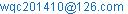(Wang Qingchao)

Wang Qingchao, Song Wanqing, Liang Jiankai. Tire Modal Analysis of ANSYS and Hypermesh Under Co-simulation.Science Discovery.Vol.3, No. 5, 2015, pp. 37-43. doi: 10.11648/j.sd.20150305.11

Abstract: The paper is aimed to analyze and get a further understanding of the dynamic characteristics of the tire by means of computing modal analysis. A tire modal testing system is built on the basis of the previous studies. We also attempt to study how the amplitude of exciting force and loading changes affect the tire modal test, which lays a foundation for applying the tire modal test into practical use. Besides, the relations of experimental modal and computing modal will also be analyzed. The analysis and application of the tire modal lay a solid foundation for medium-high frequency tire mechanical model which is being established by our research group. And it takes advantage of HYPERMESH and ANSYS to set up the finite element model of the tire and to analyze the tire structural dynamics, laying a foundation for setting, recognizing the correctness of experimental modal parameter as well as studying the relationship between calculating modal and experimental modal. And it also studies the law of tire’s dynamic characteristics and its reason. The third part makes a summary of the full text.

Keywords: Tire Modal Analysis, Hypermesh, ANSYS, Structural Dynamics

1. Introduction

Automobile tire is an important component of the car, whose structural parameters and mechanical properties of the tire determine the car's main drive performance. In a hundred years of automotive development, the studies on tire mechanics have greatly attracted researchers' attention who works in automobile field .

Vertical force longitudinal force, lateral force and moment are the result of the tire deformation, tire pressing strongly ground. Uneven pavement, the unevenness of the movement axle and tire inhomogeneity will cause the tire vibration . This vibration is transmitted to the axle and further to the vehicle body. It will lead to changes in vertical force response, which will bring important and often adversely affected to longitudinal force on the axle, lateral force and thus movement of the vehicle .

With the development of mechanics, computers and other related technologies, people have been able to create complex and accurate vehicle dynamics model, and make a simulation study of specific issues. However, in the vehicle dynamics model, practicality and accuracy of the tire model is still an important and key issue to be set. In the study of the tire model, using model methods have been noticed by domestic and foreign researchers. We can make use of modal parameters to estimate the parameters of the tire modal, and we can get the modal parameters by analyzing it.

The thesis is divided into three steps: First it summarizes the present situation of research on the dynamic tire characteristics. Then the author use HYPERMESH and ANSYS to set up the finite element model of the tire and analyze the tire structural dynamics, which aims to understand the dynamic characteristics of the tire and establish the modal test system for tires. Finally the author also studies the dynamic characteristics of the tire rule and analyzes its causes.

2. The Principle of Modal Analysis

Modal analysis can be defined as analytical analysis of structural dynamic characteristic and experimental analysis. And dynamic characteristics of the structure are characterized with the modal parameters. In mathematics, the modal parameters are eigenvalues and vectors of mechanical system differential equations of motion, that is, if you want to know the geometry boundary conditions and material properties of the structure, it’s necessary to show the distribution of mass, stiffness and damping distributions of the structure with the use of mass matrix, stiffness matrix and damping matrix. So that there is enough information to determine the modal parameters of the system (natural frequency, damping and mode shapes). It proves in theory, modal parameters can fully describe the dynamics of the system . With the studying development of modal analysis, modal analysis technique has been broadly interpreted to include determining the dynamic characteristics of the mechanical system and its application in most areas related from recognition of the system to the structure sensitivity analysis and dynamic modification, etc.

3. FEM Modal Analysis Theory

Finite element calculation mode, in fact, is structural dynamics of the eigenvalue. Eigenvalues and eigenvectors is natural frequencies and mode shapes modal analysis. Vibration system equations of motion :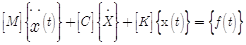(1)

[M], [C], [K], {f(t)}, {x(t)} are the mass matrix, damping matrix, stiffness matrix, force vectors and response vector respectively.

Assuming free vibration and ignoring damping: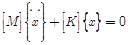(2)

This is an Order Linear Homogeneous differential equations, in the form of its solution are :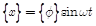(3)

Then put (3) into (2), and get :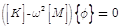(4)

Generalized eigenvalue problem, Solving this equation and that the solution can be determined features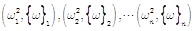, Wherein the feature value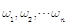represents n natural frequency of the system, and include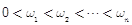, feature vector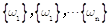represents the system of n inherent vibration mode, Their amplitude can be normalized in the following formula for quality planning: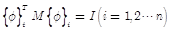(5)

Then what obtained is vector regular modes.

4. The General Structure of the Program for FEM to Solve the Problem

When using ANSYS to analyze the modal of the tire, it contains three steps: pre-processing, computation and post-processing. Pre-treatment is to change continuous actual structure into ideal mathematical model according to calculation purposes with discrete work, and forming the calculation data file in the end . Modeling follows:

1) Lead a two-dimensional model of the tire section in HYPERMESH; Clear and divide the model; set unit sets, and export it as inp format file (which can be identified by ANSYS).

2) Determine additional properties, including material parameters, boundary conditions and loads and so on.

3) Calculation and analysis. Calculate and analyze ANSYS solution module and form results file. After the calculations completed, continue to use ANSYS to deal with calculation results. Then each mode of vibration mode can be seen exactly, and provide evidence for identifying the correctness of the modal parameters of experimental modal analysis. Finite element analysis of the flowchart is shown in Fig. 1.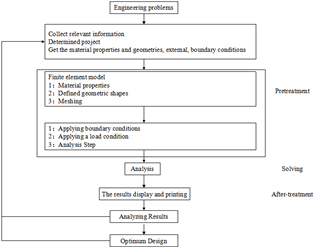Fig. 1. Finite element analysis flowchart.

5. Extract Tire Natural Frequency Extraction in ANSYS

The thesis applies the Block Lanczos method. Note that: Density must be defined in modeling; only use linear units and linear materials; non-linear nature is ignored. The specific progress of tire finite element model   in ANSYS can be found in literature. This article extracts natural frequency based on the existing finite element model of tire. In order to simplify calculation process, it’s necessary to simplify properly 3D CAD model based on the computer's performance  .

6. Tire Calculate Modal Analysis Results

Modal Characteristics of tires directly reflects the structure of the mechanical characteristics of the tire. The modal analysis of the tire enables us not only to understand the dynamics of the tire, but also provide a reference for the next tire modal experiments, such as in Modal experiment, According to modes (finite element results), we can determine whether it’s the main mode, and avoid exciting at the nodes. On the basis of the following three-dimensional finite element model of the tire, we can use Block Lanczos modal analysis techniques to simulate vibration response of the actual structure of the tire.

7. Meshing the Tire in HYPERMESH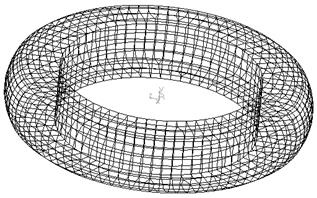Fig. 2. Mesh in HYPERMESH.

In order to directly make an analysis of the tire modal in ANSYS, this thesis deals with relatively the model that needed to be analyzed by means of the powerful pre-processing capabilities of HPERMESH. As shown in Fig. 2.

1) Frequency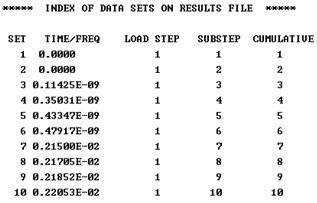Fig. 3. One to ten natural frequency.

2) Vibration pattern map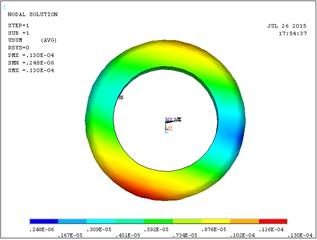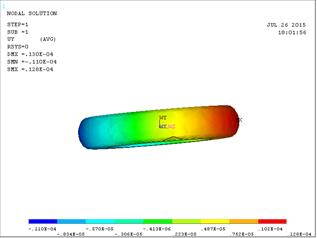Fig. 4. First-order mode (Radial and Axial).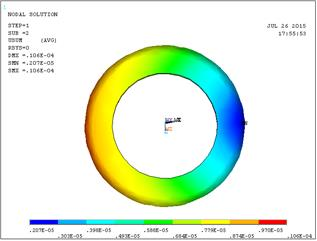Fig. 5. Second-order modes (Radial and Axial).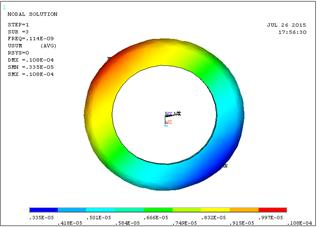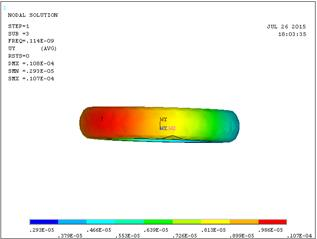Fig. 6. Third-order modes (Radial and Axial).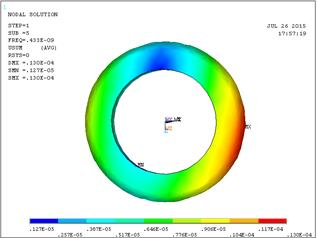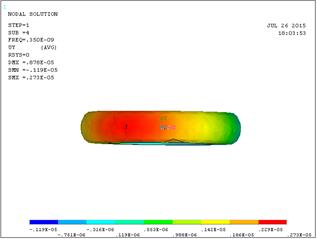Fig. 7. Fourth-order modes (Radial and Axial).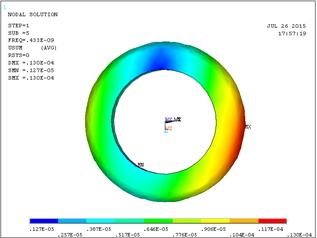Fig. 8. Fifth-order modes (Radial and Axial).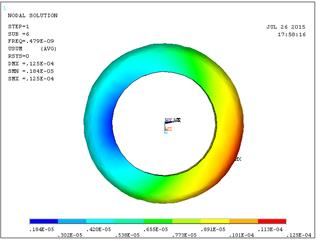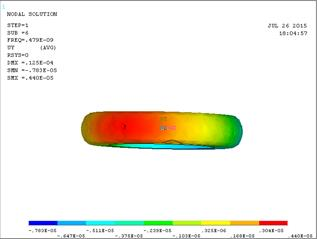Fig. 9. Sixth-order modes (Radial and Axial).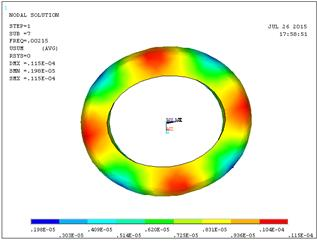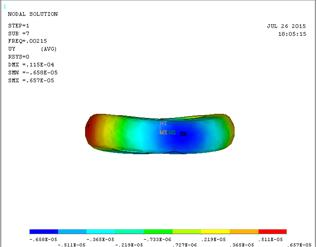Fig. 10. Seventh-order modes (Radial and Axial).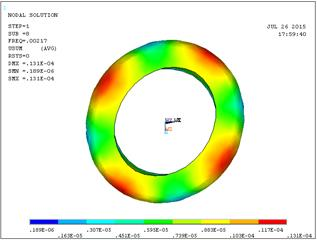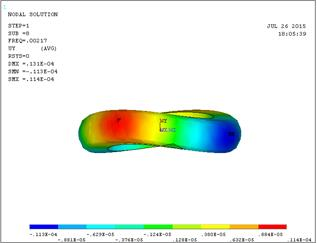Fig. 11. Eighth-order modes (Radial and Axial).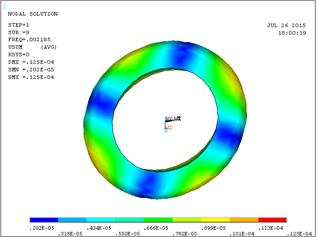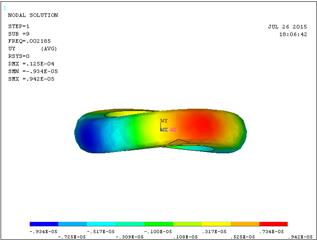Fig. 12. Ninth-order modes (Radial and Axial).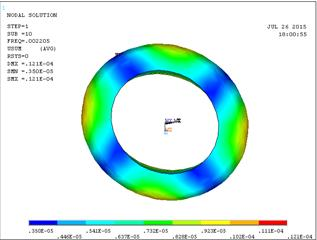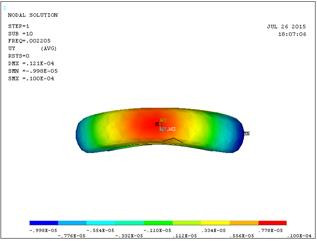Fig. 13. Tenth-order modes (Radial and Axial).

These results show that tires from the seventh stage began to show increasing twist, which explained that the design and the use of tire need to avoid these natural frequencies. It should be noted that the Dmax and Smax in the modal analysis results of displacement cloud are similar to static analysis. However, the modal (displacement) vector is a relative value, therefore it does not represent the actual physical displacement, merely reflecting the ratio between different parts of the deformation that is a kind of modal vector normalization.

8. Conclusion Analysis

Above ten order axial of the tire and radial vibration mode diagram, the conclusions can be made from the above figure:

The inherent characteristics of the tire consists of the natural frequency, mode shape modal parameters etc. It is determined by the tire itself (mass and stiffness distribution), which has nothing to do with the external load, but determine the influence of the structure on the dynamic load.

The tire vibration model case can be seen by modal analysis: tire surrounding has a large deformation. From the eighth to tenth modal, the center has maximum deformation: Frequency is 0.2105E-02, and the maximum deformation is 0.131E-04; then peripheral deformation gradually increases; in small deformation frequencies, resonance effect is not strong and big deformation results from a resonance.

So when designing a tire, we should depend on the data from modal analysis. Let the wheel natural frequency stagger relative external frequency, so it can increase comfort.

References

1. Xin zhen-Xiang, Deng Tao, Wang wei. modern tire structure design [M] Beijing: Chemical Industry Press, 2011.
2. Yu Qi,Zhou Feng,Ding Jianping. Performance and structure of the pneumatic tire[M]Guangzhou,South China University of Technology Press,1998.
3. Liu Lian-bo. All steel radial truck tire bead Performance Analysis and Improvement of[MS Thesis]Harbin Institute of Technology,2005.
4. Guan Di-hua,Wu Wei-dong,Zhang Ai-qian.Tire modeling forvertical properties by using experimental modal parameters[J].SAE,980252:17-20.
5. Jaesang Cho, Seunghwan Lee, Hyun-Yong Jeong.Finite element analysis of a tire using an equivalent cord model[J].Finite Elements in Analysis and Design,Vol 105, pp. 26-32, 2015.
6. X.Peng.G.Guo,N.Zhao.An anisotropic hyperelastic constitutive model with shear interaction for cord-rubber composites,Compos.Sci.Technol,Vol 78,pp.69-74,2013.
7. H.Li,C.Schindler.Three-dimensional finite element and analytical modelling of tyre-soil interaction,Proc.Inst.Mech.Eng.Park K[J].Multi-body Dyn,Vol 227,pp.42-60,2013
8. Domscheit A,Rother H,Winkelmann T.Refined methods for tire computation[J].Tire Science and Technology,1989,17(4):291-304.
9. V. Alkan, S.M. Karamihas, G. Anlas Finite element modeling of static tire enveloping characteristics Int. J. Automot. Technol., 12 (4), pp. 529–535,2011.
10. X. W. Yang, S. G. Zuo, and L. Lei, et al. Dynamics stability analysis of tire’s non-linear self-excited vibration[J]. China Mechanical Engineering, 2009, 20 (10): 1251-1254. (In Chinese)
11. C. Lee,J.K. Hedrick and K. Yi."Real-time slip-based estimation of maximum tire-road friction coefficient,"IEEE/ASME transaction on Mechatronics,2nd ed.,Vol 9,pp.454-458,2004.
12. Saintier N,CailletaudG,Piques R. Crack initiation and propagation under multiaxial fatigue in a natural rubberfj], International Journal of Fatigue,28(1),pp. 61-72,2006.
13. F. Yu and Y. Lin,Vehicle system dynamics.Beijing: China Machine Press,2005.
14. D. Lee, S. Kim, K. Sung, J. Park, T. Lee, S. Huh A study on the fatigue life prediction of tire belt-layers using probabilistic method J. Mech. Sci. Technol., 27 (3) (2013), pp. 673–678
15. G. Yanjin, Z. Guoqun, C. Gang3-Dimensional non-linear FEM modeling and analysis of steady-rolling of radial tires J. Reinf. Plast. Compos., 30 (3) (2011), pp. 229–240.

 Contents 1. 2. 3. 4. 5. 6. 7. 8.
Article ToolsAbstractPDF(3623K)